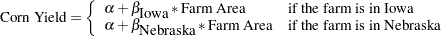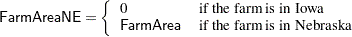## Example 90.4 Stratified Sampling

This example illustrates the use of the SURVEYREG  procedure to perform a regression in a stratified sample design. Consider a population of 235 farms producing corn in Nebraska and Iowa. You are interested in the relationship between corn yield (CornYield) and total farm size (FarmArea).

Each state is divided into several regions, and each region is used as a stratum. Within each stratum, a simple random sample with replacement is drawn. A total of 19 farms is selected by using a stratified simple random sample. The sample size and population size within each stratum are displayed in Table 90.9.

Table 90.9 Number of Farms in Each Stratum

Number of Farms

Stratum

State

Region

Population

Sample

1

Iowa

1

100

3

2

2

50

5

3

3

15

3

4

1

30

6

5

2

40

2

Total

235

19

Three models for the data are considered:

• Model I — Common intercept and slope:• Model II — Common intercept, different slope:• Model III — Different intercept and different slope:Data from the stratified sample are saved in the SAS data set Farms. In the data set Farms, the variable Weight represents the sampling weight. In the following DATA step, the sampling weights are the reciprocals of selection probabilities:

```data Farms;
input State \$ Region FarmArea CornYield Weight;
datalines;
Iowa     1 100  54 33.333
Iowa     1  83  25 33.333
Iowa     1  25  10 33.333
Iowa     2 120  83 10.000
Iowa     2  50  35 10.000
Iowa     2 110  65 10.000
Iowa     2  60  35 10.000
Iowa     2  45  20 10.000
Iowa     3  23   5  5.000
Iowa     3  10   8  5.000
Iowa     3 350 125  5.000
Nebraska 1 130  20  5.000
Nebraska 1 245  25  5.000
Nebraska 1 150  33  5.000
Nebraska 1 263  50  5.000
Nebraska 1 320  47  5.000
Nebraska 1 204  25  5.000
Nebraska 2  80  11 20.000
Nebraska 2  48   8 20.000
;
```

The information about population size in each stratum is saved in the SAS data set StratumTotals:

```data StratumTotals;
input State \$ Region _TOTAL_;
datalines;
Iowa     1 100
Iowa     2  50
Iowa     3  15
;
```

Using the sample data from the data set Farms and the control information data from the data set StratumTotals, you can fit Model I by using PROC SURVEYREG  with the following statements:

```title1 'Analysis of Farm Area and Corn Yield';
title2 'Model I: Same Intercept and Slope';
proc surveyreg data=Farms total=StratumTotals;
strata State Region / list;
model CornYield = FarmArea / covB;
weight Weight;
run;
```

Output 90.4.1 displays the data summary and stratification information fitting Model I. The sampling rates are automatically computed by the procedure based on the sample sizes and the population totals in strata.

Output 90.4.1 Data Summary and Stratum Information Fitting Model I
 Analysis of Farm Area and Corn Yield Model I: Same Intercept and Slope

The SURVEYREG Procedure

Regression Analysis for Dependent Variable CornYield

Data Summary
Number of Observations 19
Sum of Weights 234.99900
Weighted Mean of CornYield 31.56029
Weighted Sum of CornYield 7416.6

Design Summary
Number of Strata 5

Fit Statistics
R-square 0.3882
Root MSE 20.6422
Denominator DF 14

Stratum Information
Stratum
Index
State Region N Obs Population Total Sampling Rate
1 Iowa 1 3 100 3.00%
2   2 5 50 10.0%
3   3 3 15 20.0%
4 Nebraska 1 6 30 20.0%
5   2 2 40 5.00%

Output 90.4.2 displays tests of model effects and the estimated regression coefficients.

Output 90.4.2 Estimated Regression Coefficients and the Estimated Covariance Matrix
Tests of Model Effects
Effect Num DF F Value Pr > F
Model 1 21.74 0.0004
Intercept 1 4.93 0.0433
FarmArea 1 21.74 0.0004

 Note: The denominator degrees of freedom for the F tests is 14.

Estimated Regression Coefficients
Parameter Estimate Standard Error t Value Pr > |t|
Intercept 11.8162978 5.31981027 2.22 0.0433
FarmArea 0.2126576 0.04560949 4.66 0.0004

 Note: The denominator degrees of freedom for the t tests is 14.

Covariance of Estimated Regression Coefficients
Intercept FarmArea
Intercept 28.300381277 -0.146471538
FarmArea -0.146471538 0.0020802259

Alternatively, you can assume that the linear relationship between corn yield (CornYield) and farm area (FarmArea) is different among the states (Model II). In order to analyze the data by using this model, you create auxiliary variables FarmAreaNE and FarmAreaIA to represent farm area in different states:The following statements create these variables in a new data set called FarmsByState and use PROC SURVEYREG  to fit Model II:

```data FarmsByState;
set Farms;
if State='Iowa' then do;
FarmAreaIA=FarmArea;
FarmAreaNE=0;
end;
else do;
FarmAreaIA=0;
FarmAreaNE=FarmArea;
end;
run;
```

The following statements perform the regression by using the new data set FarmsByState. The analysis uses the auxiliary variables FarmAreaIA and FarmAreaNE as the regressors:

```title1 'Analysis of Farm Area and Corn Yield';
title2 'Model II: Same Intercept, Different Slopes';
proc surveyreg data=FarmsByState total=StratumTotals;
strata State Region;
model CornYield = FarmAreaIA FarmAreaNE / covB;
weight Weight;
run;
```

Output 90.4.3 displays the fit statistics and parameter estimates. The estimated slope parameters for each state are quite different from the estimated slope in Model I. The results from the regression show that Model II fits these data better than Model I.

Output 90.4.3 Regression Results from Fitting Model II
 Analysis of Farm Area and Corn Yield Model II: Same Intercept, Different Slopes

The SURVEYREG Procedure

Regression Analysis for Dependent Variable CornYield

Fit Statistics
R-square 0.8158
Root MSE 11.6759
Denominator DF 14

Estimated Regression Coefficients
Parameter Estimate Standard Error t Value Pr > |t|
Intercept 4.04234816 3.80934848 1.06 0.3066
FarmAreaIA 0.41696069 0.05971129 6.98 <.0001
FarmAreaNE 0.12851012 0.02495495 5.15 0.0001

 Note: The denominator degrees of freedom for the t tests is 14.

Covariance of Estimated Regression Coefficients
Intercept FarmAreaIA FarmAreaNE
Intercept 14.511135861 -0.118001232 -0.079908772
FarmAreaIA -0.118001232 0.0035654381 0.0006501109
FarmAreaNE -0.079908772 0.0006501109 0.0006227496

For Model III, different intercepts are used for the linear relationship in two states. The following statements illustrate the use of the NOINT option in the MODEL statement associated with the CLASS statement to fit Model III:

```title1 'Analysis of Farm Area and Corn Yield';
title2 'Model III: Different Intercepts and Slopes';
proc surveyreg data=FarmsByState total=StratumTotals;
strata State Region;
class State;
model CornYield = State FarmAreaIA FarmAreaNE / noint covB solution;
weight Weight;
run;
```

The model statement includes the classification effect State as a regressor. Therefore, the parameter estimates for effect State present the intercepts in two states.

Output 90.4.4 displays the regression results for fitting Model III, including parameter estimates, and covariance matrix of the regression coefficients. The estimated covariance matrix shows a lack of correlation between the regression coefficients from different states. This suggests that Model III might be the best choice for building a model for farm area and corn yield in these two states.

However, some statistics remain the same under different regression models—for example, Weighted Mean of CornYield. These estimators do not rely on the particular model you use.

Output 90.4.4 Regression Results for Fitting Model III
 Analysis of Farm Area and Corn Yield Model III: Different Intercepts and Slopes

The SURVEYREG Procedure

Regression Analysis for Dependent Variable CornYield

Fit Statistics
R-square 0.9300
Root MSE 11.9810
Denominator DF 14

Estimated Regression Coefficients
Parameter Estimate Standard Error t Value Pr > |t|
State Iowa 5.27797099 5.27170400 1.00 0.3337
State Nebraska 0.65275201 1.70031616 0.38 0.7068
FarmAreaIA 0.40680971 0.06458426 6.30 <.0001
FarmAreaNE 0.14630563 0.01997085 7.33 <.0001

 Note: The denominator degrees of freedom for the t tests is 14.

Covariance of Estimated Regression Coefficients
State Iowa State Nebraska FarmAreaIA FarmAreaNE
State Iowa 27.790863033 0 -0.205517205 0
State Nebraska 0 2.8910750385 0 -0.027354011
FarmAreaIA -0.205517205 0 0.0041711265 0
FarmAreaNE 0 -0.027354011 0 0.0003988349